PEAK-Rules/Predicates UserPreferences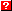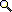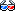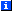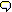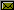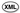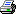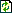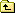# Predicate Dispatch

Here, we document and test the internals of PEAK-Rules' predicate dispatch implementation. Specifically, how it assembles the things we've already seen in the AST Building, Code Generation, Criteria and Indexing documents, into a complete implementation.

# Predicate Expression Types

Predicate expression types wrap expressions to specify what kind of dispatching should be done on the base expression. For example, predicates.IsInstance indicates that an expression is to be looked up by what it's an instance of.

There are five built-in expression types:

```>>> from peak.rules.predicates import \
...     Truth, Identity, Comparison, IsSubclass, IsInstance
```

And we will test them using code objects:

```>>> from peak.util.assembler import Code, Const, dump
>>> from dis import dis
```

## Truth

The Truth predicate tests whether its subject expression is true or false, and selects the appropriate sub-node from a (true_node, false_node) tuple:

```>>> c = Code()
>>> c(Truth(42))
>>> dump(c.code())
UNPACK_SEQUENCE          2
JUMP_IF_TRUE            L1
ROT_THREE
L1:     POP_TOP
ROT_TWO
POP_TOP
```

The generated code unpacks the 2-tuple, and then does a bit of stack manipulation to select the correct subnode.

The disjuncts() of a Truth Test is the Test itself:

```>>> from peak.rules.core import disjuncts
>>> from peak.rules.criteria import Test, Signature, Value

>>> disjuncts(Test(Truth(88), Value(True)))
[Test(Truth(88), Value(True, True))]

>>> disjuncts(Test(Truth(88), Value(True, False)))
[Test(Truth(88), Value(True, False))]
```

## Identity

The Identity predicate looks up the id() of its subject expression in a dictionary of sub-nodes. If the id isn't found, the None entry is used:

```>>> c = Code()
>>> c(Identity(99))
>>> dump(c.code())
CALL_FUNCTION            1
DUP_TOP
COMPARE_OP               6 (in)
JUMP_IF_FALSE           L1
POP_TOP
ROT_TWO
BINARY_SUBSCR
JUMP_FORWARD            L2
L1:     POP_TOP
POP_TOP
BINARY_SUBSCR
```

## Comparison

The Comparison predicate expects its "arg" to be an (exact, ranges) pair, such as might be generated by the peak.rules.indexing.split_ranges function:

```>>> c = Code()
>>> c(Comparison(555))
>>> dis(c.code())
0           0 LOAD_CONST               1 (<function value_check at ...>)
9 CALL_FUNCTION            2
```

The generated code simply calls a helper function, value_check, with its expression and argument. The helper function looks up and returns the appropriate subnode, first by trying for an exact match, and then looking for a range match if no exact match is found:

```>>> from peak.rules.predicates import value_check
>>> from peak.util.extremes import Min, Max

>>> exact = {'x':1, 'y':2}
>>> ranges = [((Min,'x'),42), (('x','y'),99), (('y',Max),88)]

>>> for letter in 'wxyz':
...     print value_check(letter, (exact, ranges))
42
1
2
88
>>> value_check('xx', (exact, ranges))
99
```

## IsSubclass

The IsSubclass predicate uses a (cache, lookup) node pair, where cache is a dictionary from classes to nodes, and lookup is a function to call with the class, in the event that the target class isn't found in the cache:

```>>> c = Code()
>>> c(IsSubclass(Const(int)))
>>> dump(c.code())
SETUP_EXCEPT            L1
DUP_TOP
UNPACK_SEQUENCE          2
ROT_THREE
POP_TOP
BINARY_SUBSCR
ROT_TWO
POP_TOP
POP_BLOCK
JUMP_FORWARD            L3
L1:     DUP_TOP
COMPARE_OP              10 (exception match)
JUMP_IF_FALSE           L2
POP_TOP
POP_TOP
POP_TOP
POP_TOP
UNPACK_SEQUENCE          2
POP_TOP
ROT_TWO
CALL_FUNCTION            1
JUMP_FORWARD            L3
L2:     POP_TOP
END_FINALLY
```

## IsInstance

The IsInstance predicate is virtually identical to IsSubclass, except that it first obtains the __class__ or type() of its target:

```>>> c = Code()
>>> c(IsInstance(Const(999)))
>>> dump(c.code())
SETUP_EXCEPT            L1
DUP_TOP
ROT_TWO
POP_TOP
POP_BLOCK
JUMP_FORWARD            L3
L1:     DUP_TOP
COMPARE_OP              10 (exception match)
JUMP_IF_FALSE           L2
POP_TOP
POP_TOP
POP_TOP
POP_TOP
ROT_TWO
CALL_FUNCTION            1
JUMP_FORWARD            L3
L2:     POP_TOP
END_FINALLY
L3:     SETUP_EXCEPT            L4
DUP_TOP
UNPACK_SEQUENCE          2
ROT_THREE
POP_TOP
BINARY_SUBSCR
ROT_TWO
POP_TOP
POP_BLOCK
JUMP_FORWARD            L6
L4:     DUP_TOP
COMPARE_OP              10 (exception match)
JUMP_IF_FALSE           L5
POP_TOP
POP_TOP
POP_TOP
POP_TOP
UNPACK_SEQUENCE          2
POP_TOP
ROT_TWO
CALL_FUNCTION            1
JUMP_FORWARD            L6
L5:     POP_TOP
END_FINALLY
```

## Defining New Predicate Types

A predicate type must be a peak.util.assembler.nodetype, capable of generating its own lookup code. The code will be used in a SMIGenerator context (see the Code Generation manual), so SMIGenerator.ARG will contain a lookup node.

Each predicate type must be usable with the predicates.predicate_node_for function, and the predicates.always_testable function:

predicate_node_for(builder, expr, cases, remaining_exprs, memo)
Return a dispatch tree node argument appropriate for the expr. The return value(s) of this function will be in the SMIGenerator.ARG local variable when the predicate type's bytecode is executed.
always_testable(expr)
Return true if the expression can always be tested, regardless of its position among the signature condition(s). Most predicate types should just implement this by calling always_testable() recursively on their target expression, and in fact all of the built-in predicate types do this. For more details, see the section on Order Independence below.

# Predicate Parsing

The CriteriaBuilder class can be used to parse Python expressions into tests and signatures. It's initialized using the same arguments as the codegen.ExprBuilder class:

```>>> from peak.rules.predicates import CriteriaBuilder, Comparison, istype
>>> from peak.rules.criteria import Disjunction, Value, Test, Range, Class, OrElse
>>> from peak.util.assembler import Local

>>> builder = CriteriaBuilder(
...     dict(x=Local('x'), y=Local('y')), locals(), globals(), __builtins__
... )
>>> pe = builder.parse

>>> pe('x+42 > 23*2')
Test(Comparison(Add(Local('x'), Const(42))), Range((46, 1), (Max, 1)))
```

The in operator converts constant classes and istype() expressions into IsInstance tests:

```>>> pe('x in int')
Test(IsInstance(Local('x')), Class(<type 'int'>, True))

>>> pe('x not in int')
Test(IsInstance(Local('x')), Class(<type 'int'>, False))

>>> pe('x in istype(int)')
Test(IsInstance(Local('x')), istype(<type 'int'>, True))

>>> pe('x not in istype(int)')
Test(IsInstance(Local('x')), istype(<type 'int'>, False))

>>> pe('x in istype(int, False)')
Test(IsInstance(Local('x')), istype(<type 'int'>, False))

>>> pe('x not in istype(int, False)')
Test(IsInstance(Local('x')), istype(<type 'int'>, True))
```

Iterable constants into or-ed equality tests:

```>>> pe('x in (1,2,3)') == Disjunction([
...     Test(Comparison(Local('x')), Value(2, True)),
...     Test(Comparison(Local('x')), Value(3, True)),
...     Test(Comparison(Local('x')), Value(1, True))
... ])
True

>>> pe('x not in (1,2,3)') == Test(
...     Comparison(Local('x')),
...     Disjunction([
...         Range((Min, -1), (1, -1)), Range((1, 1), (2, -1)),
...         Range((2, 1), (3, -1)), Range((3, 1), (Max, 1))
...     ])
... )
True
```

And non-iterable constants into plain expressions:

```>>> pe('x in 27')
Test(Truth(Compare(Local('x'), (('in', Const(27)),))), Value(True, True))

>>> pe('x not in 27')
Test(Truth(Compare(Local('x'), (('not in', Const(27)),))), Value(True, True))
```

The is operator produces identity tests, if either side is a constant:

```>>> pe('x is 42')
Test(Identity(Local('x')), IsObject(42, True))

>>> pe('42 is not x')
Test(Identity(Local('x')), IsObject(42, False))
```

And plain expressions when neither side is constant:

```>>> pe('x is y')
Test(Truth(Compare(Local('x'), (('is', Local('y')),))), Value(True, True))

>>> pe('x is not y')
Test(Truth(Compare(Local('x'), (('is not', Local('y')),))), Value(True, True))

>>> pe('not (x is y)')
Test(Truth(Compare(Local('x'), (('is', Local('y')),))), Value(True, False))

>>> pe('not (x is not y)')
Test(Truth(Compare(Local('x'), (('is not', Local('y')),))), Value(True, False))
```

Complex logical expressions are always rendered in disjunctive normal form, with negations simplified away or reduced to match flags on criteria objects:

```>>> pe('x in int and y in str')
Signature([Test(IsInstance(Local('x')), Class(<type 'int'>, True)),
Test(IsInstance(Local('y')), Class(<type 'str'>, True))])

>>> pe('not(x not in int or y not in str)')
Signature([Test(IsInstance(Local('x')), Class(<type 'int'>, True)),
Test(IsInstance(Local('y')), Class(<type 'str'>, True))])

>>> pe('x in int and (y in str or y in unicode)')
OrElse([Signature([Test(IsInstance(Local('x')),
Class(<type 'int'>, True)),
Test(IsInstance(Local('y')),
Class(<type 'str'>, True))]),
Signature([Test(IsInstance(Local('x')),
Class(<type 'int'>, True)),
Test(IsInstance(Local('y')),
Class(<type 'unicode'>, True))])])

>>> pe('not (x in int or y in str)')
Signature([Test(IsInstance(Local('x')), Class(<type 'int'>, False)),
Test(IsInstance(Local('y')), Class(<type 'str'>, False))])

>>> pe('not( x not in int and y not in str)') == OrElse([
...     Test(IsInstance(Local('x')), Class(int)),
...     Test(IsInstance(Local('y')), Class(str))
... ])
True

>>> pe('not( x in int and y in str)')
OrElse([Test(IsInstance(Local('x')), Class(<type 'int'>, False)),
Test(IsInstance(Local('y')), Class(<type 'str'>, False))])
```

And arbitrary expressions are handled as truth tests:

```>>> pe('x')
Test(Truth(Local('x')), Value(True, True))

>>> pe('not x')
Test(Truth(Local('x')), Value(True, False))
```

Note, by the way, that backquotes are not allowed in predicate expressions, as they are reserved for use by macros or "meta functions" to create specialized syntax:

```>>> pe('`x`')
Traceback (most recent call last):
...
SyntaxError: backquotes are not allowed in predicates
```

## Pattern Matching

Arbitrary expressions can be pattern matched for conversion into signatures. At the moment, the only patterns matched are isinstance and issubclass calls where the second argument is a constant, and type(x) is y expressions where y is a constant:

```>>> from peak.rules.criteria import Test, Signature, Conjunction

>>> pe('isinstance(x,int)')
Test(IsInstance(Local('x')), Class(<type 'int'>, True))

>>> pe('isinstance(x,(str,unicode))') == Disjunction([
...     Test(IsInstance(Local('x')), Class(str)),
...     Test(IsInstance(Local('x')), Class(unicode))
... ])
True

>>> pe('type(x) is int')
Test(IsInstance(Local('x')), istype(<type 'int'>, True))

>>> pe('str is not type(x)')
Test(IsInstance(Local('x')), istype(<type 'str'>, False))

>>> pe('not isinstance(x,(int,(str,unicode)))') == Test(
...     IsInstance(Local('x')), Conjunction([
...         Class(unicode, False), Class(int, False), Class(str, False)])
... )
True

>>> pe('isinstance(x,(int,(str,unicode)))') == Disjunction([
...     Test(IsInstance(Local('x')), Class(str)),
...     Test(IsInstance(Local('x')), Class(int)),
...     Test(IsInstance(Local('x')), Class(unicode))
... ])
True

>>> pe('issubclass(x,int)')
Test(IsSubclass(Local('x')), Class(<type 'int'>, True))

>>> pe('issubclass(x,(str,unicode))') == Disjunction([
...     Test(IsSubclass(Local('x')), Class(str)),
...     Test(IsSubclass(Local('x')), Class(unicode))
... ])
True

>>> pe('issubclass(x,(int,(str,unicode)))') == Disjunction([
...     Test(IsSubclass(Local('x')), Class(str)),
...     Test(IsSubclass(Local('x')), Class(int)),
...     Test(IsSubclass(Local('x')), Class(unicode))
... ])
True

>>> pe('not issubclass(x,(int,(str,unicode)))') == Test(
...     IsSubclass(Local('x')), Conjunction([
...         Class(unicode, False), Class(int, False), Class(str, False)])
... )
True

>>> pe('issubclass(int, object)')
True
```

## "Meta Function" Expansion

To create special functions with the ability to manipulate the compile-time representation of a rule, you can register "meta functions" with the meta_function decorator. You begin by defining a stub function which will be imported and used by the caller in their rules:

```>>> def let(**kw):
...     """This is a function that will have special behavior in rules"""
...     raise NotImplementedError("`let` can only be used in rules")
```

Then, you define a "meta function" for this function, that will be called at compile time. The signature of this function must match the signature with which it will be called, except that it can have zero or more extra parameters at the beginning named __builder__, __star__ and/or __dstar__. __builder__, for example, will be the active ExpressionBuilder:

```>>> def compile_let(__builder__, **kw):
...     __builder__.bind(kw)
...     return True
```

(Note: the above is not the actual implementation of the peak.rules.let() pseudo-function; the actual implementation uses a lower-level interface that allows the keywords to be seen in definition order, so this is just a demo to illustrate the operation of the meta_function decorator.)

To register your "meta function", you use @meta_function(stub_function):

```>>> from peak.rules.predicates import meta_function
>>> compile_let = meta_function(let)(compile_let)
```

Then, when the stub function is used in a rule, the meta function is called with the PEAK-Rules AST objects resulting from compiling the invocation of the stub function in the rule:

```>>> builder = CriteriaBuilder(
...     dict(x=Local('x'), y=Local('y')), locals(), globals(), __builtins__
... )
>>> pe = builder.parse

>>> pe('let(q=x*y) and q>42')
Test(Comparison(Mul(Local('x'), Local('y'))), Range((42, 1), (Max, 1)))
```

As you can see, our compile_let meta-function bound q to Mul(Local('x'), Local('y')), which was the compiled form of the keyword argument it received.

Notice, by the way, that our meta-function does NOT accept ** arguments:

```>>> pe('let(**{"z":x*y}) and z>42')
Traceback (most recent call last):
...
TypeError: <function compile_let at ...> does not support parsing **kw
```

Or * arguments::

```>>> pe('let(*[1,2]) and z>42')
Traceback (most recent call last):
...
TypeError: <function compile_let at ...> does not support parsing *args
```

This is because we didn't include __star__ or __dstar__ parameters at the beginning of the compile_let() parameter list; if we had, the function would have received either the compiled AST for the corresponding part of the call, or None if no star or double-star arguments were provided.

### Dynamic Arguments

Notice, by the way, that __star__ and __dstar__ refer to the caller's use of * and ** to make dynamic calls. The meta function can have * and ** parameters, but these are passed any static positional or keyword arguments used by the caller. For example:

```>>> def dummy(*args, **kw):
...     """Just a dummy"""

>>> def compile_dummy(__star__, __dstar__, p1, p2=None, *args, **kw):
...     print "p1        =", p1
...     print "p2        =", p2
...     print "args      =", args
...     print "kw        =", kw
...     print "__star__  =", __star__
...     print "__dstar__ =", __dstar__
...     return True

>>> compile_dummy = meta_function(dummy)(compile_dummy)

>>> builder = CriteriaBuilder(
...     dict(x=Local('x'), y=Local('y')), locals(), globals(), __builtins__
... )
>>> pe = builder.parse

>>> pe('dummy(x, y, x*x, y*y, k1=x, k2=y, *x+1, **y*2)')
p1        = Local('x')
p2        = Local('y')
args      = (Mul(Local('x'), Local('x')), Mul(Local('y'), Local('y')))
kw        = {'k2': Local('y'), 'k1': Local('x')}
__dstar__ = Mul(Local('y'), Const(2))
True

>>> pe('dummy(x)')
p1        = Local('x')
p2        = None
args      = ()
kw        = {}
__star__  = None
__dstar__ = None
True
```

### Argument Errors

Static argument errors, such as failure to pass the right number of positional arguments, and duplicate keyword arguments that occur in the source (as opposed to runtime * or ** problems), are detected at compile time:

```>>> pe('dummy(x, p1=y)')
Traceback (most recent call last):
...
TypeError: Duplicate keyword p1 for <... compile_dummy at ...>

>>> pe('dummy(p2=x, p2=y)')
Traceback (most recent call last):
...
TypeError: Duplicate keyword p2 for <... compile_dummy at ...>

>>> pe('dummy()')
Traceback (most recent call last):
...
TypeError: Missing positional argument p1 for <... compile_dummy at ...>

>>> pe('let(x)')
Traceback (most recent call last):
...
TypeError: Too many arguments for <... compile_let at ...>
```

Also, note that meta functions cannot have packed-tuple arguments:

```>>> meta_function(lambda x,y:None)(lambda x,(y,z): True)
Traceback (most recent call last):
...
TypeError: Meta-functions cannot have packed-tuple arguments
```

### Custom Argument Compiling

On occasion, a meta function may wish to interpret one or more of its arguments using a custom expression builder in place of the standard one, so that instead of a PEAK-Rules AST, it gets some other data structure. You can do this by passing keyword arguments to @meta_function() that supply a builder function for each argument that needs custom building.

A builder function is a 2-argument callable that will be passed the active ExpressionBuilder instance and the raw Python AST tuples of the argument it is supposed to parse. The function must then return whatever value should be used as the parsed form of the argument supplied to the meta function.

For example:

```>>> def make_builder(text):
...     def builder_function(old_builder, arg_node):
...         return text
...     return builder_function

>>> def dummy2(*args, **kw):
...     """Just another dummy"""

>>> compile_dummy = meta_function(dummy2,
...     p1=make_builder('p1'), p2=make_builder('p2'),
...     args=make_builder('args'), kw=make_builder('kw'),
...     k2=make_builder('k2'),
...     __star__ = make_builder('*'), __dstar__=make_builder('**')
... )(compile_dummy)

>>> builder = CriteriaBuilder(
...     dict(x=Local('x'), y=Local('y')), locals(), globals(), __builtins__
... )
>>> pe = builder.parse

>>> pe('dummy2(x, y, x*x, y*y, k1=x, k2=y, *x+1, **y*2)')
p1        = p1
p2        = p2
args      = ('args', 'args')
kw        = {'k2': 'k2', 'k1': 'kw'}
__star__  = *
__dstar__ = **
True
```

As you can see, build functions are selected on the basis of the argument name they target. If the meta function has a * parameter, each of the overflow positional arguments is parsed with the builder function of the corresponding name. If a named keyword argument has a build function, that one is used, otherwise any build function for the ** parameter is used.

### Binding Scope

Note that bindings defined by meta-functions (e.g. our let example) cannot escape "or" or "not" clauses in an expression:

```>>> pe('let(q=1) or x>q')
Traceback (most recent call last):
...
NameError: q

>>> pe('not let(q=1) and x<q')
Traceback (most recent call last):
...
NameError: q
```

But they can work within an overall not clause, as long as there are only and operators between the function call and the place where the bindings are used:

```>>> pe('not (let(q=1) and x<q)')
Test(Comparison(Local('x')), Range((1, -1), (Max, 1)))
```

Note that whenever an "or" is encountered or a "not" clause is completed, any previous bindings in effect are restored. So in this example x<q becomes x<2, since that's the binding that was in effect before the or clause:

```>>> pe('let(q=2) and (not let(q=3) or x<q)')
Test(Comparison(Local('x')), Range((Min, -1), (2, -1)))
```

### Method Argument Binding

Any bindings defined in an expression can be converted into arguments for the function associated with the rule that defined the bindings., by having its first positional argument be a named argument tuple:

```>>> from peak.rules import abstract, when, around, let
>>> def f(x): pass
>>> f = abstract(f)

>>> def dummy((q,z), x):
...     print "Got q =", q, "and z =", z
...     print "x was", x

>>> when(f, "let(q=x*2, z='whatever') and True")(dummy)
<function dummy ...>

>>> f(42)
Got q = 84 and z = whatever
x was 42
```

This even works when you have a next_method argument after the tuple, and even if your method is defined inside a closure:

```>>> def closure(y):
...     def dummy2((a,b,c), next_method, x):
...         print "a, b, c =", (a,b,c)
...         print "y was", y
...         return next_method(x)
...     return dummy2

>>> around(f, "let(a='a', b=x*3, c=b+23)")(closure(99))
<function dummy2 ...>

>>> f(42)
a, b, c = ('a', 126, 149)
y was 99
Got q = 84 and z = whatever
x was 42
```

At the moment, this actually works by recalculating the expressions in a wrapper function that then invokes your original method, so it's more of a DRY thing than an efficiency thing. That is, it keeps you from accidentally getting your rule and your function out of sync, and saves on retyping or copy-pasting.

(Future versions of PEAK-Rules, however, may improve this so that the bindings aren't recalculated at every method level, or perhaps aren't recalculated at all. It's tricky, though, because depending on the calculations involved, it might be more efficient to redo them than to do the dynamic stack inspection that would be needed to locate the active expression cache! So, in that event, the main value would be supporting at-most-once execution of expressions with side-effects.)

# Custom Predicate Functions

The @expand_as decorator lets you specify a string that will be used in place of a function, when the function is referenced in a condition.

Here's a trivial example:

```>>> from peak.rules import expand_as, value

>>> def just(arg): pass

>>> expand_as("arg")(just)
<function just ...>

>>> def f(x): pass

>>> when(f, "x==just(42)")(value(23))
value(23)

>>> f(42)
23
```

In the above, the just(arg) function is defined as being the same as its argument. So, the "x==just(42) is treated as though you'd just said "x==42".

And, although we never defined an actual implementation of the just() function, it actually still works:

```>>> just(42)
42
```

This is because if you decorate an empty function with @expand_as, the supplied condition will be compiled and attached to the existing function object for you. (This saves you having to actually write the body.)

Of course, if you decorate, say, an already-existing function that you want to replace, then nothing happens to that function:

```>>> def isint(ob):
...     print "called!"
...     return isinstance(ob, int)

>>> expand_as("isinstance(x, int)")(isint)
<function isint ...>

>>> isint(42)
called!
True
```

But, the correct expansion still happens when you use that function in a rule:

```>>> around(f, "isint(x)")(value(99))
value(99)

>>> f(42)
99
```

Note that it's ok to use let() or other binding-creating expressions inside an expansion string, and they won't interfere with the surrounding conditions:

```>>> def oddment(a, b): pass

>>> expand_as("let(x=a*2, y=x+b+1) and y")(oddment)
<function oddment ...>

>>> oddment(27, 51)  # prove bindings work even in a function
106

>>> around(f, "x==oddment(27, 51) and x==106 and isint(x)")(value('yeah!'))
value('yeah!')

>>> f(106)  # prove that x doesn't get redefined after oddment
'yeah!'
```

In the above, temporary variables x and y are created in the expansion, but they don't affect the original value of x in the rule where the function is expanded.

Of course, this also means that you can't implement something like a pattern-matching feature or the let() function using @expand_as. It's just an easier way to handle the sort of common cases where meta-functions would be overkill.

# Expression to Predicate Conversion

Meta-functions are only one way to transform a Python expression into a predicate. It's also possible to register somewhat-arbitrary transformations by registering methods with the expressionSignature() generic function.

In this section, we'll create a simple "priority" predicate that doesn't influence method selection, but affects implication order between predicates.

The basic idea is that we'll create a priority type that's an integer subclass, and use it in expressions of the form isisntance(foo, Bar) and priority(3), that will then have precedence over an identical expression with a lower priority:

```>>> from peak.rules import when
>>> from peak.rules.core import implies

>>> class priority(int):
...     """A simple priority"""

>>> when(implies, (priority, priority))(lambda p1,p2: p1>p2)
<function <lambda> ...>

>>> implies(priority(3), priority(2))
True

>>> implies(priority(2), priority(3))
False
```

To use our new type, we'll need to implement a conversion from a Const(somepriority) expression, to a Test(priority, somepriority) condition.

Normally, these conversions are handled by the expressionSignature() generic function in the peak.rules.predicates module.

By default, expressionSignature() simply takes the expression object it's given, and returns Test(Truth(expr), Value(True)) -- that is, a truth test on the boolean value of the expression. Or, if the value given is a constant, it simply returns an immediate boolean value:

```>>> from peak.rules.predicates import expressionSignature

>>> expressionSignature(Const(priority(3)))
True
```

So, we need to register a method that handles priorities appropriately:

```>>> when(expressionSignature, "expr in Const and expr.value in priority")(
...     lambda expr: Test(None, expr.value)
... )
<function <lambda> ...>
```

Okay, let's try out our new condition:

```>>> from peak.rules import value

>>> def dummy(arg): return "default"
>>> when(dummy, "arg==1 and priority(1)")(value("1 @ 1"))
value('1 @ 1')

>>> dummy(1)
'1 @ 1'
>>> dummy(2)
'default'

>>> when(dummy, "arg==1 and priority(2)")(value("1 @ 2"))
value('1 @ 2')

>>> dummy(1)
'1 @ 2'
>>> dummy(2)
'default'

>>> when(dummy, "arg==2 and priority(2)")(value("2 @ 2"))
value('2 @ 2')

>>> dummy(1)
'1 @ 2'
>>> dummy(2)
'2 @ 2'

>>> when(dummy, "arg==2 and priority(1)")(value("2 @ 1"))
value('2 @ 1')

>>> dummy(1)
'1 @ 2'
>>> dummy(2)
'2 @ 2'
```

In order to allow a function to safely upgrade from type-only dispatch to full predicate dispatch, it's necessary for predicate engines to support using type tuples as signatures (since such tuples may already be registered with the function's RuleSet).

To support this, the tests_for() function takes an optional second parameter, representing the engine that "wants" the tests, and whose argument names will be used to accomplish the conversion:

```>>> from peak.rules.core import Dispatching, implies
>>> from peak.rules.criteria import tests_for
>>> engine = Dispatching(implies).engine

>>> list(tests_for((int,str), engine))
[Test(IsInstance(Local('s1')), Class(<type 'int'>, True)),
Test(IsInstance(Local('s2')), Class(<type 'str'>, True))]

>>> list(tests_for((istype(tuple),), engine))
[Test(IsInstance(Local('s1')), istype(<type 'tuple'>, True))]
```

Each element of the type tuple is converted using a second generic function, type_to_test:

```>>> from peak.rules.predicates import type_to_test

>>> type_to_test(int, Local('x'), engine)
Test(IsInstance(Local('x')), Class(<type 'int'>, True))

>>> type_to_test(istype(str), Local('x'), engine)
Test(IsInstance(Local('x')), istype(<type 'str'>, True))

>>> class x: pass
>>> type_to_test(x, Local('x'), engine)
Test(IsInstance(Local('x')), Class(<class ...x at ...>, True))
```

If you implement a new kind of class test for use in type tuples, you'll need to add the appropriate method(s) to type_to_test if you want it to also work with the predicate engine.

So, let's test the actual upgrade process, and also confirm that you can still pass in type tuples (or precomputed tests, signatures, etc.) after upgrading:

```>>> def demo(ob): pass
>>> tmp = when(demo, (int,))(value('int'))
>>> tmp = when(demo, (str,))(value('str'))

>>> demo(42)
'int'

>>> demo('test')
'str'

>>> tmp = when(demo, "ob in int and ob==42")(value('Ultimate answer'))
>>> tmp = when(demo, (list,))(value('list'))
>>> tmp = when(demo, Test(IsInstance(Local('ob')), Class(tuple)))(
...     value('tuple')
... )

>>> demo(42)

>>> demo([])
'list'

>>> demo(())
'tuple'

>>> demo('test'), demo(23)
('str', 'int')
```

And, just for the heck of it, let's make sure that you can upgrade to an IndexedEngine by using any other values on a TypeEngine function:

```>>> def demo(ob): pass
>>> tmp = when(demo, Test(IsInstance(Local('ob')), Class(tuple)))(
...     value('tuple')
... )

>>> demo(())
'tuple'
```

# Criterion Ordering

Criterion ordering for a predicate dispatch engine is defined by the ordering of the tests in its signatures. Any test expression that is not defined as always_testable, must not be computed until after any test expressions to its left have been tested. But tests whose expression is just a local variable (i.e., a plain function argument), do not have such restrictions:

```>>> from peak.rules.predicates import IndexedEngine
>>> from peak.rules import abstract, when
>>> from peak.rules.indexing import Ordering

>>> def f(a,b): pass
>>> f = abstract(f)
>>> m = when(f, "isinstance(a, int) and a+b==42")(value(None))
>>> engine = Dispatching(f).engine
>>> list(Ordering(engine, IsInstance(Local('a'))).constraints)
[frozenset([])]
[frozenset([IsInstance(Local('a'))])]

>>> def f(a,b): pass
>>> f = abstract(f)
>>> m = when(f, "isinstance(b, str) and a+b==42 and isinstance(a, int)")(
...     value(None)
... )
>>> engine = Dispatching(f).engine
>>> list(Ordering(engine, IsInstance(Local('a'))).constraints)
[frozenset([])]
>>> list(Ordering(engine, IsInstance(Local('b'))).constraints)
[frozenset([])]
[frozenset([IsInstance(Local('b'))])]

>>> def f(a,b): pass
>>> f = abstract(f)
>>> m = when(f, "isinstance(a, int) and isinstance(b, str) and a+b==42")(
...     value(None)
... )
>>> engine = Dispatching(f).engine

>>> list(Ordering(engine, IsInstance(Local('a'))).constraints)
[frozenset([])]

>>> list(Ordering(engine, IsInstance(Local('b'))).constraints)
[frozenset([])]

>>> try: frozenset and None
... except NameError: from peak.rules.core import frozenset

>>> list(
... ) == [frozenset([IsInstance(Local('a')), IsInstance(Local('b'))])]
True
```

## Order Independence

The determination of whether a test expression can be used in an order- independent way, is via the always_testable() function:

```>>> from peak.rules.predicates import always_testable
```

In general, only locals and constants can have their tests applied independent of signature ordering:

```>>> always_testable(Local('x'))
True

>>> always_testable(Const(99))
True

False
```

And predicate test expressions are evaluated according to their tested expression:

```>>> always_testable(IsInstance(Local('x')))
True
False

>>> always_testable(Comparison(Local('x')))
True
False

>>> always_testable(Identity(Local('x')))
True
False

>>> always_testable(Truth(Local('x')))
True
False
```

Except for IsSubclass(), which may need to have other tests applied before it:

```>>> always_testable(IsSubclass(Local('x')))
False
```

If you create a new predicate type, be sure to define a method for always_testable that will recursively invoke always_testable on the predicate's target expression. If you don't do this, then your predicate type will always be treated as order-dependent, even if its target expression is a local or constant.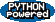EditText of this page (last modified 2011-08-31 21:12:05) FindPage by browsing, title search , text search or an index Or try one of these actions: AttachFile, DeletePage, LikePages, LocalSiteMap, SpellCheck